1. Back To Blog

# Exception Handling in C# using example step by step

## What is an exception?

Exception is an event or an unwanted behavior which disrupts the normal of the application.

Exception handling features help you deal with any unexpected situation that occurs when a program is running. Exceptions are unforeseen things that happen in a program.

Most of the time, you can detect and handle program errors in code and sometimes you cannot. For example, you cannot predict when you'll receive a file I/O error, run out of system memory, or database error. In the below example, a method test for division by zero and catch the error. Without the exception handling this, program would terminate with a DivideByZeroException.

```using System;
using System.Collections.Generic;
using System.Linq;
using System.Text;
namespaceexceptionhandling

classexceptionhandling

staticvoid Main(string[] args)

Console.WriteLine(Divide(10, 0));
Console.WriteLine("Rahul");
}
privatestaticint Divide(int number1, int number2)

int number3 = number1 / number2;
return number3;
}
}
}
```

OUTPUT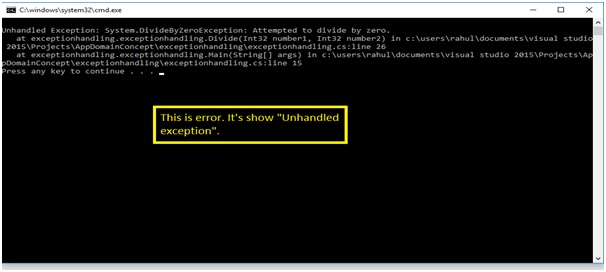I would suggest you to visit our c# step by step site and watch this you tube video on exception handling which explain C# exceptions in more details https://www.youtube.com/watch?v=9jmA0UO45-Q

## Handling exception using try, catch, finally and throw.

Exception provide a way to transfer control from one part of a programto another. Exception handling is built upon keywords try, catch, finally and throw.

Try: A try block identifies a block of code for which particular exceptions is activated. It is followed by one or more catch blocks.

Catch: A program catches an exception with an exception handler at the place in a program where you want to handle the problem. The catch keyword indicates the catching of an exception.

Finally: The finally block is used to execute a given set of statements, whether an exception is thrown or not thrown. For example, if you open a file, it must be closed whether an exception is raised or not.

Throw: A program throws an exception when a problem shows up. This is done using a throw keyword.

```using System;
using System.Collections.Generic;
using System.Linq;
using System.Text;
namespaceexceptionhandling

classexceptionhandling

staticvoid Main(string[] args)

try

Console.WriteLine(Arthmetic(10, 0));
}
catch(Exception ex)

Console.WriteLine(ex.Message);
}
finally

Console.WriteLine("thank you");
}
}
privatestaticintArthmetic(int No1, int No2)
//and then arthmetic throw the exception to "main function" Exception is handl

int value = Divide(No1, No2);
return value;
}
privatestaticint Divide(int No1, int No2)
//When any error in divide, it throw the exception to Arthmetic function

if ((No1<0) || (No2 < 0))

thrownewException("This is the Negative number");
}
int number3 = No1 / No2;
return number3;
}
}
}```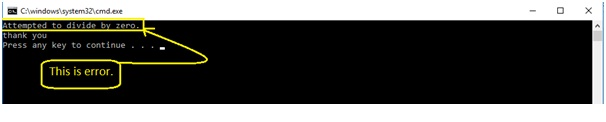## Exception propagation

An exception occurs in a try block, the corresponding catch blocks are checked to see if they can catch the exception.

The main function is calling arithmetic function and then Arithmetic function is calling Divide function. Then any exception occurs in divide first throw the exception to arithmetic function and then arithmetic function throw the exception to the main function. Basically, in c# are propagation to the caller.

```using System;
using System.Collections.Generic;
using System.Linq;
using System.Text;
namespaceexceptionhandling

classexceptionhandling

staticvoid Main(string[] args)

try

Console.WriteLine(Arithmetic(-1, 0));
}
catch (DivideByZeroException ex) // child class
//Divide Exception

Console.WriteLine("  Divide by zero is not possible corrected to 1");
Console.WriteLine(Arithmetic(10, 1));
}
catch (Exception ex)
// this exception is a parent class
// normal exception handling

Console.WriteLine(ex.InnerException);
}
finally

Console.WriteLine("  thank you");
}
}
privatestaticint Arithmetic(int No1, int No2)
//and then arthmetic throw the exception to "main function" Exception is handl

int value = Divide(No1, No2);
return value;
}
privatestaticint Divide(int No1, int No2)
//When any error in divide, it throw the exception to Arthmetic function

if ((No1 < 0) || (No2 < 0))

thrownewException("This is the Negative number");
}
int number3 = No1 / No2;
return number3;
}
}
}```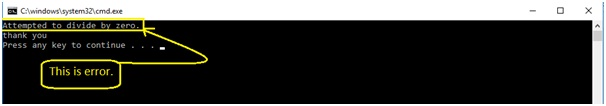If no matching exception is found even if a finally block is executed the exception is propagated to a higher-level try block. This process repeats until the exception is caught but if it is not caught, the program execution comes to an end. In other words, if the top of the call stack is reached without finding a catch block handling the exception, the default exception handler handles it and then the application terminates.

## Multiple catch

A single try statement can contain multiple conditional catch blocks, each of which handles a specific type of exception.

You have a multiple catch statement, you can treat each, one of these exception in a separate way.

```using System;
using System.Collections.Generic;
using System.Linq;
using System.Text;
namespaceexceptionhandling

classexceptionhandling

staticvoid Main(string[] args)

try

Console.WriteLine(Arthmetic(10, 0));
}
catch(DivideByZeroException ex)

Console.WriteLine("Divide by zero is not possible corrected to 1");
Console.WriteLine(Arthmetic(10, 1));
}
catch (Exception ex)

Console.WriteLine(ex.Message);
}
finally

Console.WriteLine("thank you");
}
}
privatestaticintArthmetic(int No1, int No2)
//and then arthmetic throw the exception to "main function" Exception is handl

int value = Divide(No1, No2);
return value;
}
privatestaticint Divide(int No1, int No2)
//When any error in divide, it throw the exception to Arthmetic function

if ((No1<0) || (No2 < 0))

thrownewException("This is the Negative number");
}
int number3 = No1 / No2;
return number3;
}
}
}
```

OUTPUT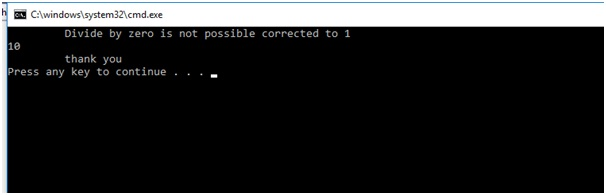When the pass the negative value it's the error.

```using System;
using System.Collections.Generic;
using System.Linq;
using System.Text;
namespaceexceptionhandling

classexceptionhandling

staticvoid Main(string[] args)

try

Console.WriteLine(Arithmetic(-10, 0)); // when the negative value ,
}
catch (DivideByZeroException ex) // child class
//Divide Exception

Console.WriteLine("  Divide by zero is not possible corrected to 1");
Console.WriteLine(Arithmetic(10, 1));
}
catch (Exception ex)
// this exception is a parent class
// normal exception handling

Console.WriteLine(ex.StackTrace);// stackTrece told about the holecall heppenand which layer is problem.
}
finally

Console.WriteLine("  thank you");
}
}
privatestaticint Arithmetic(int No1, int No2)
//and then arthmetic throw the exception to "main function" Exception is handl

int value = Divide(No1, No2);
return value;
}
privatestaticint Divide(int No1, int No2)
//When any error in divide, it throw the exception to Arthmetic function

if ((No1 < 0) || (No2 < 0))

thrownewException("This is the Negative number");
}
int number3 = No1 / No2;
return number3;
}
}
}
```

Output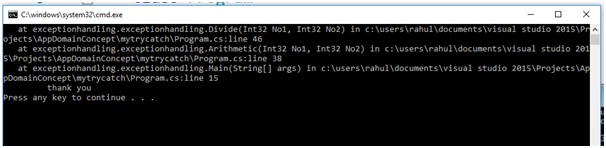## Important of multiple exception

1. Not all catch block are executed when some exception occurs. Only the best matched catch block will get executed.
2. From the name of the exception classes their purpose should be easily understood.
3. 3. If some other type of exception occurs in the code apart from the type of exceptions which are mentioned above, then the code block 8 will be get executed. This is because it does not correspond to some specific type of error. It is capable to handling all types of errors because its arguments are of the type Exception.

Creating custom exception class

```using System;
using System.Collections.Generic;
using System.Linq;
using System.Text;
namespaceexceptionhandling

classexceptionhandling

staticvoid Main(string[] args)

try

Console.WriteLine(Arithmetic(1, 1));
}
catch (NumDenoEqualException ex) // child class
//Divide Exception, handling Sparely

Console.WriteLine(1);
}
catch (DivideByZeroException ex) // child class
//Divide Exception

Console.WriteLine("  Divide by zero is not possible corrected to 1");
Console.WriteLine(Arithmetic(10, 1));
}
catch (Exception ex)
// this exception is a parent class
// normal exception handling

Console.WriteLine(ex.InnerException);
}
finally

Console.WriteLine("  thank you");
}
}
privatestaticint Arithmetic(int No1, int No2)
//and then arthmetic throw the exception to "main function" Exception is handl

int value = Divide(No1, No2);
return value;
}
privatestaticint Divide(int No1, int No2)
//When any error in divide, it throw the exception to Arthmetic function

if ((No1 < 0) || (No2 < 0))

thrownewException("This is the Negative number");
}
if ((No1 == No2))

thrownewNumDenoEqualException("Numerator and Denomert0r");
}
int number3 = No1 / No2;
return number3;
}
publicclassNumDenoEqualException : Exception

publicNumDenoEqualException(string message) : base(message)

}
}
}
}
```

output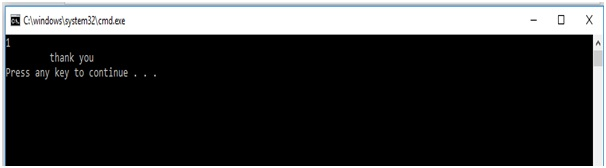when we pass the negative value in try (-1,1) then it's show that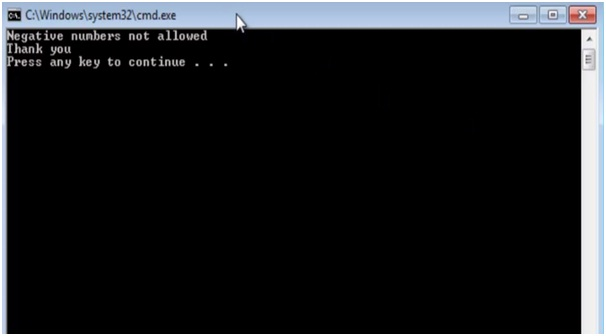If we pass the value in the try (-1,0) then, we get system exception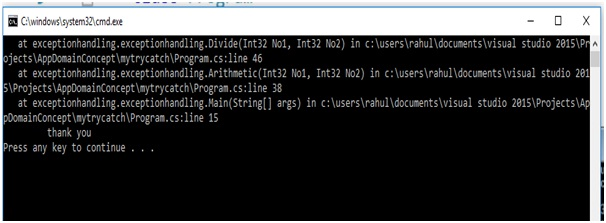## Creating User-Defined Exceptions

You can also define your own exception. User-defined exception classes are derived from the Exception class.

```using System;
using System.Collections.Generic;
using System.Linq;
using System.Text;
namespaceexceptionhandling

classexceptionhandling

staticvoid Main(string[] args)

try

Console.WriteLine(Arithmetic(10, 0)); // when the negative value ,
}

catch (Exception ex)
// this exception is a parent class
// normal exception handling

Console.WriteLine(ex.Message);
//stackTrece told about the hole call heppen and which layer is problem
}
finally

Console.WriteLine("  thank you");
}
}
privatestaticint Arithmetic(int No1, int No2)
//and then arthmetic throw the exception to "main function" Exception is handl

int value = Divide(No1, No2);
return value;
}
privatestaticint Divide(int No1, int No2)
//When any error in divide, it throw the exception to Arthmetic function

if ((No1 < 0) || (No2 < 0))

//user define exception
thrownewException("This is the Negative number");
}
int number3 = No1 / No2;
return number3;
}
}
}
```

OUTPUT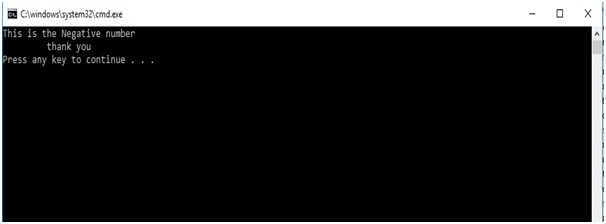Watch our latest video on C# interview questions & answers  :-Visit us @ www.questpond.com or call us at 022-66752917... read more#### .NET interview questions 6th edition (Sixth edition) - By Shivprasad Koirala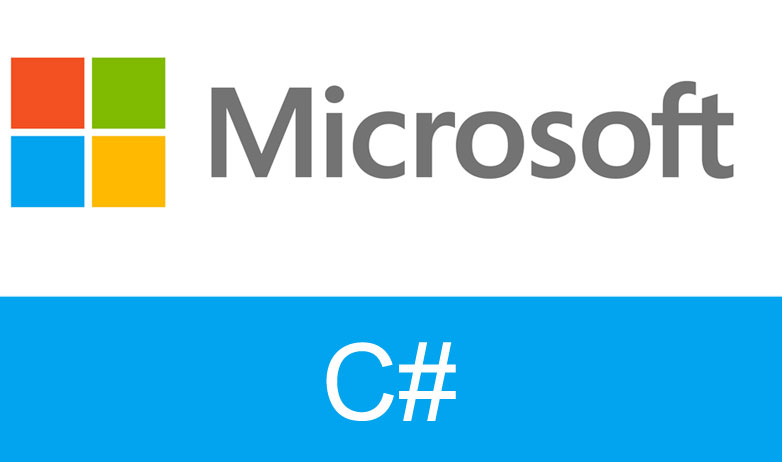#### C# Design pattern interview questions with answers: - How Singleton is different from Static class?#### Exception Handling in C# using example step by step#### MVC vs ASP.NET ( MVC Interview question with answers )?#### What is the difference between each version of MVC 2, 3, 4, 5 and 6? (MVC Interview Questions)